Definitions of Square Dance Calls and Concepts

Index -->  Plus  |  A1  |  A2  |  C1  |  C2  |  C3A  |  C3B  |  C4  |  NOL  |
Definitions (Text Only) -->  Plus  |  A1  |  A2  |  C1  |  C2  |  C3A  |  C3B  |  C4  |  NOL  |
 Find call:

 C3A definitions from theCeder Chest of C3.This material is copyrighted, so please treat it accordingly.For complete explanations, diagrams and examples, please purchase the book.To view a definition, click the desired link.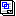C3A list1/4 (or 3/4) Mix

\$B1&\$N(B Mini-Wave \$B\$N(B Box, Center \$B\$,1&\$N(B Mini-Wave \$B\$N(B Diamond, \$B\$^\$?\$OB>\$NE,@Z\$J(B formation \$B\$+\$i!%(B

1. \$B\$G\$-\$k?M\$,(B Right Arm Turn 1/4 (\$B\$^\$?\$O(B 3/4) \$B\$r\$7(B;
2. Line \$B\$+(B Wave \$B\$N?M\$,(B Centers Cross Run \$B\$r\$7\$^\$9!%(B;
3. Line \$B\$+(B Wave \$B\$N?M\$,(B Centers Trade \$B\$r\$7\$^\$9!%(B.

Line \$B\$^\$?\$O(B wave \$B\$G=*\$o\$j\$^\$9!%(B

1/4 (\$B\$^\$?\$O(B 3/4) Mix \$B\$O(B 3 \$B%Q!<%H\$N%3!<%k\$G\$9(B.

1/4 (or 3/4) The Deucey

\$BJ?9T\$J(B Wave \$B\$+\$i!%(B

1/4 The Deucey: Arm Turn 1/4 \$B\$r\$7\$F(B, Centers \$B\$,(B Cast Off 1/4 \$B\$r\$9\$k4V\$K(B, Lead End \$B\$O(B Circulate \$B\$r(B, Trailing End \$B\$O(B 1/2 Circulate \$B\$r\$7\$F(B, Center Star \$B\$,(B turn 1/4 \$B\$r\$7(B, \$B2q\$C\$??M\$,(B (Center Wave) Cast Off 1/4 \$B\$r\$7\$F(B, lonesome Center \$B\$O(B move up (Phantom Hourglass Circulate) \$B\$r\$7(B Wave \$B\$N(B End \$B\$K\$J\$j\$^\$9!%J?9T\$J(B Wave \$B\$G=*\$o\$j\$^\$9!%(B

3/4 The Deucey: \$B3F!9\$N(B 1/4 turn \$B\$,(B 3/4 turn \$B\$K\$J\$k0J30\$O(B 1/4 The Deucey \$B\$HF1\$8\$G\$9!%(B

1/4 (or 3/4) Wheel The Ocean | Sea

1/4 Wheel The Ocean | Sea:
\$B:8\$N(B Two-Faced Line \$B\$+\$i!%(BLeft Cast 1/4 \$B\$r\$7(B (\$B8~\$+\$\$9g\$C\$?%+%C%W%k\$H\$J\$j(B), Finish Wheel The Ocean | Sea \$B\$r\$7\$^\$9!%(B(Ocean: Belles \$B\$,

3/4 Wheel The Ocean | Sea:
\$B1&\$N(B Two-Faced Line \$B\$+\$i!%(BLeft Cast 3/4 \$B\$r\$7(B (\$B8~\$+\$\$9g\$C\$?%+%C%W%k\$H\$J\$j(B), Finish Wheel The Ocean | Sea \$B\$r\$7\$^\$9!%(BMini-Wave \$B\$N(B Box \$B\$G=*\$o\$j\$^\$9!%(B

1/4 (\$B\$^\$?\$O(B 3/4) Wheel The Ocean | Sea \$B\$O(B 2 \$B%Q!<%H\$N%3!<%k\$G\$9!%(B

any Tagging call Chain Thru

1. any Tagging call \$B\$r(B 1/2 Tag \$B\$N(B position \$B\$^\$G9T\$\$(B,
2. Scoot Chain Thru.

\$BJ?9T\$J(B Wave \$B\$G=*\$o\$j\$^\$9!%(B

anything Reaction

anything call \$B\$r:G8e\$N(B Extend \$B\$NA0\$^\$G9T\$C\$F(B, Chain Reaction \$B\$rA4It9T\$\$\$^\$9!%(B

\$BIaDL(B anything call \$B\$O(B Tagging call \$B\$N(B Scoot Back \$B%P%j%(!<%7%g%s\$G\$9!%(B

\$BNc(B,
Flip Reaction: \$BJ?9T\$J(B Wave \$B\$+\$i!%(B Flip Back Centers To A Wave \$B\$r\$7(B, \$BA40w\$G(B Chain Reaction \$B\$r\$7\$^\$9!%(B
Tag Reaction: \$BJ?9T\$J(B Wave \$B\$+\$i!%(B Tag Back Centers To A Wave \$B\$r\$7(B, \$BA40w\$G(B Chain Reaction \$B\$r\$7\$^\$9!%(B
Load The Boat Reaction: From Facing Lines (or R-H Tidal Wave). Load The Boat Centers To A Wave \$B\$r\$7(B, \$BA40w\$G(B Chain Reaction \$B\$r\$7\$^\$9!%(B

Bias Circulate

\$BJ?9T\$J(B Wave \$B\$^\$?\$OB>\$NE,@Z\$J(B formation \$B\$+\$i!%(B

Leaders \$B\$O(B Circulate \$B\$r(B, Trailers \$B\$O(B Diagonal Box Circulate \$B\$r\$7\$^\$9!%(B \$BDL\$jJ}(B: \$B1&\$N(B Wave \$B\$+\$i\$O(B Trailers \$B\$O1&

\$BJ?9T\$J(B Wave \$B\$+\$i\$O(B, \$BJ?9T\$J(B Wave \$B\$G=*\$o\$j\$^\$9!%(B

Big Block CONCEPT

Interlocked Blocks, Butterfly, "O" \$B\$^\$?\$OB>\$NE,@Z\$J(B formation \$B\$+\$i!%(B

\$BM?\$(\$i\$l\$?%3!<%k\$r(B Distorted Parallel Lines \$B\$GF0\$-\$^\$9!%%3!<%k\$N=*\$o\$j\$G(B, \$B3F!9\$N?M\$O%*%j%8%J%k\$N(B formation \$B\$N0LCV(B (\$BLu

Breaker anything

Columns, Magic Columns, \$B\$^\$?\$OE,@Z\$J(B T-Bone \$B\$K\$J\$C\$F\$\$\$k(B 2 x 4 \$B\$+\$i!%(B

Ends \$B\$O(B Cast Off 3/4 \$B\$r(B, Centers \$B\$O(B Box Counter Rotate 1/4 \$B\$r\$7\$F(B 1/2 Box Circulate \$B\$r\$7\$^\$9!%(B \$B\$=\$7\$F(B, Center Line \$B\$,(B anything call (\$B\$b\$7(B anything call \$B\$,(B 1, 2, 3 \$B\$N\$h\$&\$J?t\$@\$C\$?\$i(B, 1/4 \$B\$K\$=\$N?t\$r3]\$1\$?(B Cast Off 1/4, Cast Off 2/4\$B!J(B1/2\$B!K(B, Cast Off 3/4) \$B\$r\$7(B, \$BB>\$N?M\$O(B move up (Phantom Hourglass Circulate) \$B\$r\$7\$^\$9!%(B

anything call \$B\$K\$h\$j\$^\$9\$,(B, \$BIaDL(B Parallel Lines, Twin Diamonds \$B\$^\$?\$O(B Hourglass \$B\$G=*\$o\$^\$9!%(B

Catch anything {n}

\$BE,@Z\$J(B formation \$B\$+\$i!%(B

Square Thru n To A Wave \$B\$r\$7(B, anything call \$B\$r\$7\$F(B, Step & Fold \$B\$r\$7\$^\$9!%(B

\$B\$\$\$m\$\$\$m\$J(B formations \$B\$G=*\$o\$j\$^\$9!%(B

Central Concept

anything \$B%3!<%k\$N(B centers \$B\$N%Q!<%H\$r9T\$\$\$^\$9(B.

\$B\$b\$7;O\$a\$N(B formation \$B\$,(B 2 x 4 \$B\$G(B, anything \$B%3!<%k\$N(B centers \$B\$N%Q!<%H\$,(B 1 x 4 \$B\$H(B 2 x 2 \$B\$N\$I\$A\$i\$+\$i\$G\$b=PMh\$k\$H\$-(B (\$BNc\$(\$P(B, Counter \$B\$d(B Detour) \$B\$O(B, 2 x 2 \$B\$GF0\$-\$^\$9(B. \$B\$3\$N>l9g(B, \$B%3!<%i!<\$O%@%s%5!<\$K\$I\$A\$i\$N(B setup \$B\$GF0\$/\$+(B (\$BNc\$(\$P(B, Each Wave do a Central Counter) \$B\$r;X<(\$9\$Y\$-\$G\$9(B.

Checker Board | Box anything

Each Line \$B\$K(B leader \$B\$,(B 2 \$B?M\$@\$1\$N(B 2 x 4 (\$B\$"\$k\$\$\$OB>\$NE,@Z\$J(B formation) \$B\$+\$i!%(B

\$B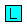\$B\$O(B each Line \$B\$N(B Leaders \$B\$r<(\$7(B,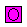\$B\$OB>\$N%@%s%5!<\$r<(\$7\$^\$9!%(B\$B\$G<(\$5\$l\$??M\$O(B, 1 x 4 \$B\$G(B\$B\$N?M\$HF1\$88~\$-\$G\$J\$1\$l\$P\$I\$3\$r8~\$\$\$F\$\$\$F\$b\$+\$^\$\$\$^\$;\$s!%(B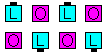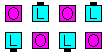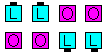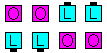Leader \$B\$,(B\$B1&\$N(B Wave \$B\$N(B setup... \$B:8\$N(B Wave \$B\$N(B setup... \$B1&\$N(B 2FL setup... \$B:8\$N(B 2FL setup.

Checker Board anything:
Each Line \$B\$N(B Leader (\$B\$N?M(B) \$B\$,\$*8_\$\$\$K(B Trade \$B\$r\$9\$k4V\$K(B, \$BB>\$N?M\$O(B anything call \$B\$r(B distorted 1 x 4 \$B\$G9T\$\$\$^\$9!%(B 2 x 4 \$B\$G=*\$o\$j\$^\$9!%(B

Checker Box anything:
Each Line \$B\$N(B Leader (\$B\$N?M(B) \$B\$,\$*8_\$\$\$K(B Trade \$B\$r\$9\$k4V\$K(B, \$BB>\$N?M\$O(B anything call \$B\$r(B distorted 2 x 2 \$B\$G9T\$\$\$^\$9!%(B 2 x 4 \$B\$G=*\$o\$j\$^\$9!%(B

Couple Up

\$BE,@Z\$J(B 2 x 2 \$B\$+\$i!%(B

Box Circulate \$B\$r\$7(B, Leaders U-Turn Back (2 x 2 \$B\$NCf?4\$NJ}\$X(B) \$B\$r\$7\$^\$9!%(B

2 x 2 \$B\$G=*\$o\$j\$^\$9!%(B

Couple Up \$B\$O(B 2 \$B%Q!<%H\$N%3!<%k\$G\$9!%(B

Cross Chain Reaction

\$BE,@Z\$J0lHL2=\$5\$l\$?(B 1/4 Tag \$B\$+\$i!%(B

Very Centers \$B\$,Counter Rotate 1/4 (\$B30B&\$r2s\$k(B) \$B\$r\$7\$F(B, each side \$B\$N(B Centers \$B\$,(B Hinge \$B\$r\$7(B, Centers \$B\$,(B Star Circulate \$B\$r\$9\$k4V\$K(B Outsides \$B\$O(B Trade \$B\$r\$7(B, each side \$B\$N(B Centers \$B\$,(B Cast Off 3/4 \$B\$r\$9\$k4V\$KB>\$N?M\$O(B Phantom Hourglass Circulate \$B\$r\$7\$^\$9!%(B

\$BJ?9T\$J(B Line \$B\$G=*\$o\$j\$^\$9!%(B

Cross Counter

3/4 Tag \$B\$^\$?\$OB>\$NE,@Z\$J(B formation \$B\$+\$i!%(B

Centers \$B\$O(B Trade The Wave, Cast Off 3/4 \$B\$r\$7(B, Counter Rotate 1/4 \$B\$r\$7\$^\$9!%30B&\$N?M\$O(B Cross Cast Back, Touch 1/2 \$B\$r\$7(B, Step & Fold \$B\$r\$7\$^\$9!%(B

\$B1&\$N(B 3/4 Tag \$B\$+\$i\$O(B, \$BJ?9T\$J:8\$N(B Two-Faced Lines \$B\$K\$J\$j(B, \$B:8\$N(B 3/4 Tag \$B\$+\$i\$O(B, \$BJ?9T\$J:8\$N(B Waves \$B\$K\$J\$j\$^\$9!%(B

Cross Counter \$B\$O(B 3 \$B%Q!<%H\$N%3!<%k\$G\$9!%(B

Delight | Dilemma

\$BE,@Z\$J(B formation (\$BIaDL\$O(B 3/4 Tag) \$B\$+\$i!%(B

Outsides \$B\$O(B 1/4 Right (Delight \$B\$N\$H\$-(B) \$B\$^\$?\$O(B 1/4 Left (Dilemma \$B\$N\$H\$-(B) \$B\$r\$7\$F(B, Circulate twice \$B\$r\$7(B, Centers \$B\$O(B Swing, Slip twice, Cast Off 3/4 \$B\$r\$7\$^\$9!%(B

Delight | Dilemma \$B\$N(B Center \$B\$N%Q!<%H\$O(B, 4 \$B%Q!<%H\$N%3!<%k\$G\$9!%(B

Drift Apart

2 x 4 \$B\$+\$i!%(B

Ends \$B\$O(B Split Circulate twice \$B\$r\$7(B, Centers \$B\$G30\$r8~\$\$\$F\$\$\$k?M\$O(B Crossover Circulate and Circulate \$B\$r\$7(B, Centers \$B\$GCf\$r8~\$\$\$F\$\$\$k?M\$O(B Circulate and Crossover Circulate \$B\$r\$7\$^\$9!%(B

2 x 4 \$B\$G=*\$o\$j\$^\$9!%(B

Drift Apart \$B\$O(B 2 \$B%Q!<%H\$N%3!<%k\$G\$9!%(B

Ease Off

\$BJ?9T\$J(B Line \$B\$^\$?\$OB>\$NE,@Z\$J(B formation \$B\$+\$i!%(B

Outsides \$B\$O(B Zing \$B\$r(B, Centers \$B\$O(B (Concentric) Circulate \$B\$r\$7(B Face In \$B\$r\$7\$^\$9!%(B

\$BJ?9T\$J(B Line \$B\$+\$i\$O(B Double Pass Thru \$B\$G=*\$o\$j\$^\$9!%(B

Eight By anything

\$BE,@Z\$J(B formation \$B\$+\$i!%(B

Outsides \$B\$O(B Grand Chain Eight and Roll \$B\$r\$7(B, Centers \$B\$O(B anything \$B\$N%3!<%k\$r\$7\$^\$9(B (Center \$B\$GF0\$-\$^\$9(B)\$B!%(B

\$B\$\$\$m\$\$\$m\$J(B formation \$B\$G=*\$o\$j\$^\$9!%(B

Exchange The Box

2 x 4 \$B\$+\$i!%(B

Split Circulate \$B\$r9g7W(B 4 \$B2s9T\$\$\$^\$9\$,(B, \$BCf\$r8~\$\$\$?(B Center \$B\$H\$J\$C\$?\$H\$-\$O\$N(B box \$B\$X(B cross over \$B\$7\$^\$9(B)\$B!%(BExchange The Box \$B\$O(B 4 \$B\$D\$N%Q!<%H\$KJ,\$1\$i\$l\$^\$9!%(B

\$BB>\$N(B box \$B\$X(B cross \$B\$9\$k\$H\$-\$NDL\$jJ}\$G\$9\$,(B, \$B\$^\$@<+J,\$N%*%j%8%J%k\$N(B box \$B\$K\$\$\$k?M\$N30B&\$rDL\$j\$^\$9!%Cf\$r8~\$\$\$?(B Centers \$B\$,(B Exchange \$B\$N%j!<%@!<\$H\$J\$j(B, \$BIaDL\$N>l9g(B box \$B\$NB>\$N?M\$O\$=\$N?M\$K\$D\$\$\$F9T\$/\$3\$H\$,\$G\$-\$^\$9!%(B

Exchange The Triangle

Twin Triangles (1 \$BBP\$N(B Triangle) \$B\$+\$i!%(B

\$B\$N(B Triangle \$B\$X(B Circulate \$B\$7\$^\$9!%(B (Apex-to-Apex Triangles \$B\$+\$i\$N(B Circulate \$B\$O(B Interlocked Triangle Circulate \$B\$H\$J\$j(B, Base-to-Base Triangles \$B\$+\$i\$N(B Circulate \$B\$OExchange The Triangle \$B\$O(B 3 \$B\$D\$N%Q!<%H\$KJ,\$1\$i\$l\$^\$9!%(B

Expand The Column

\$B30B&\$,(B Column \$B\$N(B 2 x 4 \$B\$^\$?\$OB>\$NE,@Z\$J(B formation \$B\$+\$i!%(B

Centers \$B\$O(B Phantom Run (Reverse Flip) \$B\$r(B, \$B30B&\$N?M\$O(B Column Circulate twice \$B\$r\$7\$^\$9!%(B

Columns \$B\$+\$i\$OJ?9T\$J(B Line \$B\$K\$J\$j\$^\$9!%(B

Explode The Top

\$BJ?9T\$J(B Line \$B\$+\$i!%(B

1. Explode;
2. End Beau Circulate 1 & 1/2 as End Belle Circulate, Veer inward, and U-Turn Back as Centers Touch 1/2 and the Very Centers Cast Off 3/4 while the other Center Step Ahead to form a R-H Star;
3. Turn the Star 1/4;
4. The End of the Center Wave walks forward as everyone else follows to Unwrap The Star, and everyone Face In to end in Facing Lines.

Fancy

Column \$B\$+\$i!%(B

Trailers (#2 \$B\$H(B #4 dancers) \$B\$,(B Press Out \$B\$r\$7\$F(B, Trailing Couple \$B\$O(B 1/2 Press Ahead \$B\$r\$7(B, Outsides work Phantom: all Any Shoulder Turn & Deal \$B\$r\$7\$^\$9!%(B

Double Pass Thru formation \$B\$G=*\$o\$j\$^\$9!%(B

Finally Concept

Finally anyConcept anything Anything call \$B\$rIaDL\$K;O\$a(B, \$B:G8e\$N%Q!<%H\$N\$_M?\$(\$i\$l\$?(B CONCEPT \$B\$G9T\$\$\$^\$9!%(B

Flare Out To A Line

\$BJ?9T\$J(B Two-Faced Lines \$B\$HB>\$NE,@Z\$J(B formation \$B\$+\$i!%(B

Leaders \$B\$O%;%C%H\$NCf?4\$+\$iN%\$l\$k\$h\$&\$K(B Turn To A Line \$B\$r9T\$\$(B, Trailers \$B\$O(B 1/2 Circulate \$B\$r\$7\$^\$9!%(B

\$BJ?9T\$J(B Two-Faced Lines \$B\$+\$i\$O(B As Couples Wave \$B\$G=*\$o\$j\$^\$9!%(B

Mini Wave \$B\$N(B Box \$B\$^\$?\$OE,@Z\$J(B T-Bone \$B\$N(B 2 x 2 \$B\$+\$i!%(B

\$B1&\$^\$?\$O:8\$N(B Mini Wave \$B\$N(B Box \$B\$+\$i\$O(B Diamond \$B\$G=*\$o\$j(B, T-Bone \$B\$N(B 2 x 2 \$B\$+\$i\$OIaDL(B Wave \$B\$G=*\$o\$j\$^\$9!%(B

\$BJ?9T\$J(B Wave \$B\$+\$i!%(B

Leaders \$B\$,(B 1/2 Split Circulate \$B\$r\$7\$F(B Tandem Cross Fold \$B\$r\$9\$k4V\$K(B, Trailers \$B\$O(B Follow Your Neighbor (Extend and Arm Turn 3/4) \$B\$r\$7(B, \$BA40w\$G(B Tandem Extend \$B\$r\$7\$^\$9!%(B

Column \$B\$G=*\$o\$j\$^\$9!%(B

Grand Mix

\$B0lHL2=\$5\$l\$?(B Tidal Line \$B\$+\$i!%(B

Center pairs \$B\$N?M(B (8\$B?M\$N(B Tidal Line \$B\$G\$O(B 3 pairs, 6\$B?M\$N(B Tidal Line \$B\$G\$O(B 2 pairs) \$B\$O(B, Grand (Working As Centers) Cross Run (Grand Slip-Slide \$B\$N\$h\$&\$K(B) \$B\$r\$7(B, new Center pairs \$B\$,(B Trade (Grand Slip) \$B\$r\$7\$^\$9!%(B

Tidal Line \$B\$G=*\$o\$j\$^\$9!%(B 2 \$B%Q!<%H\$N%3!<%k\$G\$9!%(B

Hinge The Lock, Lock The Hinge

Hinge The Lock:
T-Bone \$B\$G\$J\$\$E,@Z\$J(B 2 x 2 \$B\$+\$i!%(BHinge, Lockit \$B\$r\$7\$^\$9!%(BWave \$B\$G=*\$o\$j\$^\$9!%(B

Lock The Hinge:
Wave \$B\$^\$?\$O(B Two-Faced Line \$B\$+\$i!%(BLockit, Hinge \$B\$r\$7\$^\$9!%(BMini-Wave \$B\$N(B Box \$B\$G=*\$o\$j\$^\$9!%(B

\$BN>J}\$H\$b(B 2 \$B%Q!<%H\$N%3!<%k\$G\$9!%(B

anyone Hop

T-Bone \$B\$G\$O\$J\$\$E,@Z\$J(B 2 x 2 (\$BIaDL\$O8~\$+\$\$9g\$C\$?%+%C%W%k(B) \$B\$+\$i!%(B

\$B;X<(\$5\$l\$??M\$,(B Walk, \$BB>\$N?M\$,(B Dodge \$B\$r\$7\$F(B, \$BA40w\$G(B Hinge \$B\$r\$7\$^\$9!%(B

Wave \$B\$^\$?\$O(B Inverted Line \$B\$G=*\$o\$j\$^\$9!%(B 2 \$B%Q!<%H\$N%3!<%k\$G\$9!%(B

Initially Concept

Initially anyConcept anything anything \$B\$N%3!<%k\$N;O\$a\$N%Q!<%H\$r(B, \$BM?\$(\$i\$l\$?(B CONCEPT \$B\$G9T\$\$\$^\$9!%\$=\$7\$F(B, anything \$B\$N%3!<%k\$N;D\$j\$r9T\$\$\$^\$9(B (\$B\$9\$J\$o\$A(B, \$BM?\$(\$i\$l\$?(B CONCEPT \$B\$r;H\$o\$:\$K(B)\$B!%(B

Interlocked Little

\$B0lHL2=\$5\$l\$?(B 1/4 Line \$B\$^\$?\$OB>\$NE,@Z\$J(B formation \$B\$+\$i!%(B

Outsides \$B\$O(B 1/4 Right \$B\$r\$7\$F(B Counter Rotate 1/4 (\$B\$9\$J\$o\$A(B, Little) \$B\$r\$7(B, Centers \$B\$O(B Step And Cross Fold (Ends \$B\$,(B Cross Fold, Centers \$B\$O(B 1/2 Press Ahead) \$B\$r\$7\$^\$9!%(B

\$B0lHL2=\$5\$l\$?(B 1/4 Line \$B\$+\$i\$OJ?9T\$J(B Line \$B\$G=*\$o\$j\$^\$9!%(B

Interlocked Plenty

\$B0lHL2=\$5\$l\$?(B 1/4 Line \$B\$^\$?\$OB>\$NE,@Z\$J(B formation \$B\$+\$i!%(B

1. Interlocked Little (Outsides 1/4 Right and Counter Rotate 1/4 as Centers Step And Cross Fold);
2. Interlocked Little \$B\$r\$7\$F(B, Split Circulate twice (\$BIaDL(B Tandem Partner Trade \$B\$N\$h\$&\$K\$J\$j\$^\$9(B) \$B\$^\$?\$O(B Reverse The Pass \$B\$r\$7(B,
3. Outsides \$B\$O(B Counter Rotate 1/4 and Roll \$B\$r(B, Centers \$B\$O(B Concentric 1/2 Zoom \$B\$r\$7\$^\$9!%(B

This is a 3-part call.

Interlocked Scoot (Back)

1/4 Line \$B\$+\$i!%(B

\$B<+J,\$N(B Interlocked Single 1/4 Tag \$B\$N?M(B (Interlocked Diamond \$B\$N0LCV\$K\$\$\$k?M(B) \$B\$G(B Scoot Back \$B\$r\$7\$^\$9!%(B

3/4 Line \$B\$G=*\$o\$j\$^\$9!%(B

Jay Concept

\$B0lHL2=\$5\$l\$?(B 1/4 Tag \$B\$^\$?\$OB>\$NE,@Z\$J(B formation \$B\$+\$i!%(B

Jay Walk \$B\$r\$9\$k?M\$?\$A\$N(B Distorted (\$B\$f\$,\$s\$@(B) Box \$B\$GF0\$-\$^\$9!%%3!<%k\$N=*\$o\$j\$G(B, \$B\$3\$l\$i\$N(B 4 \$B\$D\$N(B footprint \$B\$N0LCV\$r:F9=@.\$7\$^\$9!%(B

Keep Busy

\$BJ?9T\$J(B Two-Faced Line \$B\$+\$i!%(B

Leader \$B\$O(B Couples Circulate With The Flow \$B\$r\$7(B, Trailers \$B\$O(B 1/2 Circulate, Very Centers Hinge, Flip The Diamond, Step & Fold \$B\$r\$7\$^\$9!%(B

\$BJ?9T\$J(B Wave \$B\$G=*\$o\$j\$^\$9!%(BTrailer \$B\$K\$H\$C\$F\$O(B 4 \$B%Q!<%H\$N%3!<%k\$G\$9!%(B

Latch On (fraction)

Tandem \$B\$N(B 2 \$B?M(B, \$B8~\$+\$\$9g\$C\$?(B 2 \$B?M(B, \$B\$"\$k\$\$\$OGXCf9g\$o\$;\$N(B 2 \$B?M\$+\$i!%(B

Right Roll To A Wave \$B\$r\$7\$F(B, Arm Turn 1/4 (\$B\$"\$k\$\$\$O;X<(\$5\$l\$?(B fraction) \$B\$r\$7\$^\$9!%(B

\$B1&\$N(B Mini-Wave \$B\$G=*\$o\$j(B, 2 \$B%Q!<%H\$N%3!<%k\$G\$9!%(B

Leaders \$B\$O30B&\$N?M\$r(B pivot point \$B\$K\$7\$F(B Cast Off 1/4 (Out Anchor 1/4) and Roll \$B\$r\$7\$F(B, Press Ahead \$B\$r\$7(B, Trailers \$B\$O(B 1/2 Circulate \$B\$r\$7\$F(B, Cross Fire \$B\$r\$7\$^\$9!%(B

\$BJ?9T\$J(B Wave \$B\$G=*\$o\$j\$^\$9!%(B

Locker's Choice

\$BJ?9T\$J(B Wave \$B\$+\$i!%(B
1. Lockit;
2. Center Wave \$B\$O(B Lockit \$B\$r(B, \$BB>\$N?M\$O(B Trade \$B\$r\$7(B,
3. Center Wave \$B\$O(B Hinge \$B\$r(B, \$BB>\$N?M\$O(B Step & Fold \$B\$r\$7\$^\$9!%(B

\$B5U\$N

Mini-Chase

\$BGXCf9g\$o\$;\$N%+%C%W%k\$^\$?\$OE,@Z\$J(B T-Bone \$B\$N(B 2 x 2 (\$BA40w\$,(B Leads) \$B\$+\$i!%(B

Belles \$B\$O(B Shakedown \$B\$r(B, Beaus \$B\$O(B Partner Tag \$B\$r9T\$\$\$^\$9!%(B

\$BGXCf9g\$o\$;\$N%+%C%W%k\$+\$i\$O(B, \$B1&\$N(B Mini Wave \$B\$N(B Box \$B\$G=*\$o\$j\$^\$9!%(B

Open Up The Column

Column \$B\$+\$i!%(B

1 \$BHVL\\$N?M\$O(B Trail Off \$B\$r\$7\$F(B Roll \$B\$r\$7(B, 2 \$BHVL\\$N?M\$O(B Circulate, Peel Off \$B\$r\$7\$F(B Roll \$B\$r\$7(B, 3 \$BHVL\\$H(B 4 \$BHVL\\$N?M\$O(B Circulate, Centers Cast Off 3/4 \$B\$r\$7(B, \$BA40w\$G(B Extend \$B\$r\$7\$^\$9!%(B

\$BJ?9T\$J(B Wave \$B\$G=*\$o\$j\$^\$9!%(B

Own The anyone anything By anything

\$B;X<(\$5\$l\$??M\$O(B, \$B;O\$a\$N(B anything call \$B\$N<+J,\$N%Q!<%H\$r9T\$\$(B, \$BB>\$N?M\$O(B, 2 \$BHVL\\$N(B anything call \$B\$N<+J,\$N%Q!<%H\$r9T\$\$\$^\$9!%(B

Patch (The) anyone

\$B%+%C%W%k\$^\$?\$O(B mini wave \$B\$+\$i!%(B

Hinge \$B\$r\$7\$F(B, \$B;X<(\$5\$l\$??M\$,(B flow \$B\$NJ}\$X(B U-Turn Back (Roll twice) \$B\$r\$7\$^\$9!%(B

\$B%+%C%W%k\$^\$?\$O(B mini wave \$B\$G=*\$o\$j\$^\$9!%\$3\$l\$O(B 2 \$B%Q!<%H\$N%3!<%k\$G\$9!%(B

\$BCm

Peel Chain Thru

Trade By \$B\$^\$?\$O(B 3/4 Tag \$B\$+\$i!%(B

Centers \$B\$O(B Arm Turn 1/2 \$B\$r\$7\$F(B Slip \$B\$r\$7(B, Outsides \$B\$O(B Cast Back \$B\$r\$7\$F(B Touch 1/2 \$B\$r\$7(B, each side \$B\$N(B centers \$B\$,(B Cast Off 3/4 \$B\$r\$7(B, \$BB>\$N?M\$O(B Fan The Top \$B\$N<+J,\$N%Q!<%H\$r\$7\$^\$9!%(B

\$B8~\$+\$\$9g\$C\$?(B Line \$B\$^\$?\$OB>\$NE,@Z\$J(B formation \$B\$+\$i!%(B

Centers \$B\$O(B Touch 1/4 \$B\$r\$7\$F(B, Cross Concentric Vertical Tag The Line \$B\$r\$7(B, Lead \$B\$N?M\$O(B Peel Left, Trailer \$B\$N?M\$O(B Peel Right \$B\$r\$7\$F%+%C%W%k\$G=*\$o\$j\$^\$9(B (Sets In Motion \$B\$N\$h\$&\$K(B)\$B!%(B \$B\$=\$N4V\$K(B Outsides \$B\$O(B Circulate once and a half, Hinge, Cross Concentric Vertical 1/2 Tag \$B\$r\$7\$F(B, Face In \$B\$r\$7\$^\$9!%(B

\$B8~\$+\$\$9g\$C\$?(B Line \$B\$+\$i\$O(B Double Pass Thru \$B\$G=*\$o\$j\$^\$9!%(B

Polly Wally

\$B0lHL2=\$5\$l\$?(B Column \$B\$+\$i!%(B

Ends \$B\$O(B, Mirror Turn To A Line (Promenade \$BJ}8~\$X(B 'Turn & Deal') \$B\$r\$7(B, Centers \$B\$O(B Leads U-Turn Back (\$B:82s\$j\$K(B), Veer Right, As Couples Extend \$B\$r\$7\$^\$9!%(B

\$BJ?9T\$J(B Line \$B\$G=*\$o\$j\$^\$9!%(B

Quick Step | anything

Quick Step [C3A]: 1/4 Box \$B\$^\$?\$OB>\$NE,@Z\$J(B formation \$B\$+\$i!%(B2 \$BHVL\\$N?M\$O(B Press Out \$B\$r\$7\$F(B, \$BNY\$N?M\$H(B Phantom Ah So \$B\$r\$7(B, \$B\$=\$NB>\$N?M\$O(B Circulate \$B\$r\$7\$^\$9!%(B1/4 Box \$B\$+\$i\$O(B Column \$B\$G=*\$o\$j\$^\$9!%(B

Quick anything [C3A]: 1/4 Box \$B\$^\$?\$O(B Column \$B\$+\$i!%(B2 \$BHVL\\$N?M\$O(B Press Out \$B\$r\$7\$F(B, \$B2q\$C\$??M\$H(B (\$BI,MW\$J\$i(B Phantom \$B\$H(B) anything \$B\$N%3!<%k\$r\$7(B, \$B\$=\$NB>\$N?M\$O(B Circulate \$B\$r\$7\$^\$9!%(B Outsides \$B\$H(B centers \$B\$N4V\$K(B Phantom \$B\$,\$G\$-\$k\$H\$-\$O(B, \$B%3!<%k\$N=*\$o\$j\$G

Rally

\$B0lHL2=\$5\$l\$?(B 1/4 Tag \$B\$^\$?\$OB>\$NE,@Z\$J(B formation \$B\$+\$i!%(B

Centers \$B\$O(B Step & Fold \$B\$r\$7\$F(B Peel & Trail \$B\$r\$7\$^\$9!%(B Outsides \$B\$O(B 1/4 Right \$B\$r\$7\$F(B, Counter Rotate 1/4 \$B\$r\$7(B, \$BCf\$X(B (Inward, Right \$B\$^\$?\$O(B Left \$B\$N(B) Roll To A Wave \$B\$r\$7\$^\$9!%(B

\$B0lHL2=\$5\$l\$?(B 1/4 Tag \$B\$+\$i\$O(B Tidal Line \$B\$G=*\$o\$j\$^\$9!%(B Centers \$B\$K\$H\$C\$F\$O(B 2 \$B%Q!<%H\$N%3!<%k\$G\$9!%(B

Reach Out

Mini-Wave \$B\$N(B Box, Tandem Couple, \$B\$^\$?\$OE,@Z\$J(B 2 x 2 \$B\$N(B T-Bone \$B\$+\$i!%(B

Trailers \$B\$O(B 1/2 Box Circulate \$B\$r\$7\$F(B, Phantom Run (Reverse Flip) \$B\$r\$7(B, Leaders \$B\$O(B Box Circulate 1 & 1/2 \$B\$r\$7\$^\$9!%(B

Mini-Wave \$B\$N(B Box \$B\$O(B Wave \$B\$H\$J\$j(B, 2 x 2 \$B\$N(B T-Bone \$B\$OIaDL(B Diamond \$B\$H\$J\$j(B, Tandem Couple \$B\$O(B One-Faced Line \$B\$H\$J\$j\$^\$9!%(B

Recoil

T-bone \$B\$G\$J\$\$E,@Z\$J(B 2 x 2 \$B\$+\$i!%(B

Mini-Wave \$B\$N(B Box \$B\$G=*\$o\$j\$^\$9!%(B2 \$B%Q!<%H\$N%3!<%k\$G\$9!%(B

Release anything

\$B0lHL2=\$5\$l\$?(B 1/4 Tag \$B\$^\$?\$O(B, \$B0lHL2=\$5\$l\$?(B 1/4 Diamond \$B\$+\$i!%(B

Center 4 \$B\$N(B ends \$B\$O(B Press Ahead \$B\$r(B, \$BNY\$K?M\$,\$3\$J\$+\$C\$?(B Outside \$B\$N?M\$O(B (12-Matrix \$B\$G(B) Press Out \$B\$r\$7\$^\$9!%(B \$B\$=\$7\$F(B, Outsides \$B\$O(B, \$B\$b\$7I,MW\$J\$i(B phantom \$B\$H9T\$\$(B, \$BA40w\$G(B anything \$B\$N%3!<%k\$r\$7\$^\$9!%(B

\$BCmanything \$B\$N%3!<%k\$,(B phantom \$B\$r4^\$`%3%s%;%W%H\$N%3!<%k\$G\$"\$k\$H\$-0J30\$O(B, Outsides \$B\$H(B centers \$B\$N4V\$N(B phantom \$B\$O%3!<%k\$N=*\$o\$j\$G\$\$\$J\$/\$J\$j\$^\$9!%(B \$BNc\$(\$P(B, Release Triple Wave Ah So \$B\$G\$O(B phantom \$B\$r;D\$7\$^\$9\$,(B, Release Ah So \$B\$G\$O\$H\$j=|\$-\$^\$9!%(B

Scatter Circulate

\$BJ?9T\$J(B Two-Faced Line \$B\$HB>\$NE,@Z\$J(B 2 x 4 formation (\$BNc\$(\$P8~\$+\$\$9g\$C\$?(B Line, \$BGXCf9g\$o\$;\$N(B Line, \$B\$=\$7\$F\$"\$k(B T-Bone) \$B\$+\$i!%(B

Ends \$B\$O(B Split Circulate \$B\$r(B, \$B30\$r8~\$\$\$?(B Centers \$B\$O(B Crossover Circulate \$B\$r(B, \$BCf\$r8~\$\$\$?(B Centers \$B\$O(B Concentric (Box) Crossover Circulate \$B\$r\$7\$^\$9!%(B

\$BJ?9T\$J(B Two-Faced Line \$B\$+\$i\$O(B, \$BJ?9T\$J(B Wave \$B\$G=*\$o\$j\$^\$9!%(B

Scoot The Diamond

Single 1/4 Tag \$B\$+\$i!%(B

Scoot Back \$B\$r\$7\$F(B, Outsides \$B\$,(B handhold (\$B\$H\$C\$F\$\$\$k

Diamond \$B\$G=*\$o\$j\$^\$9!%(B

Single Checkmate

2 x 2 \$B\$+\$i!%(B

Leaders \$B\$O(B Box Circulate twice \$B\$r\$7\$F(B 1/4 In (Box Transfer) \$B\$r(B, Trailers \$B\$O(B Box Circulate - 1/4 In - Box Circulate \$B\$r\$7\$^\$9!%(B

2 x 2 \$B\$G=*\$o\$j\$^\$9!%(B

Single Concept

Single \$B\$O(B, 8 \$B?M\$N%3!<%k\$r(B 4 \$B?M\$N%3!<%k\$K(B, \$B\$"\$k\$\$\$O(B 4 \$B?M\$N%3!<%k\$r(B 2 \$B?M\$N%3!<%k\$K\$9\$kJ}K!\$G\$9!%(B

\$B\$"\$k%3!<%k\$N(B Single \$B\$G\$O(B, \$B\$=\$N%3!<%k\$N(B 2 \$B?M\$K\$h\$C\$FDL>o9T\$o\$l\$kF0\$-\$r(B, 1 \$B?M\$G9T\$\$\$^\$9!%\$7\$?\$,\$C\$F(B, Single \$B\$,\$"\$k%3!<%k\$O(B, \$BA4\$F\$N?M\$r(B 2 \$B?M\$N=8\$^\$j\$KJ,\$1\$k\$3\$H\$,2DG=\$G(B, \$B\$=\$N(B 2 \$B?M\$O(B, \$B%3!<%k\$rF0\$/4V\$:\$C\$HF1\$8J}\$r8~\$\$\$F\$\$\$kI,MW\$,\$"\$j\$^\$9!%\$=\$N(B 2 \$B?M\$O(B, \$B%3!<%k\$N<+J,\$NItJ,\$r(B As Couples, In Tandem, Once Removed, \$B\$^\$?\$O(B Twosome (\$B\$^\$?\$O\$3\$l\$i\$N%3%s%;%W%H\$NAH\$_9g\$o\$;(B) \$B\$GF0\$-\$^\$9!%(B

Single \$B\$r(B, 2 \$B?M\$N%@%s%5!<\$N=8\$^\$j\$r(B 2 \$B?M\$N%@%s%5!<\$N??\$sCf\$K\$\$\$k(B 1 \$B?M\$N%@%s%5!<\$GCV\$-49\$(\$k(B, \$B\$H9M\$(\$k\$3\$H\$,\$G\$-\$^\$9!%(B \$B\$3\$N(B single \$B\$N%@%s%5!<\$O(B, \$B%3!<%k\$rIaDL\$KF0\$\$\$F\$\$\$k(B 2 \$B?M\$N\$A\$g\$&\$I??\$sCf\$N0LCV\$K\$\$\$D\$b\$\$\$FF0\$/\$3\$H\$K\$J\$j\$^\$9!%(B

Single File Recycle | Recoil

Single File Recycle [C3A]: Single Double Pass Thru \$B\$+\$i!%(BCenters \$B\$O(B Beaus \$B\$NLr\$r(B, Ends \$B\$O(B Belles \$B\$NLr\$r\$7\$F(B facing Recycle \$B\$r\$7\$^\$9(B (\$B\$9\$J\$o\$A(B, Centers \$B\$O(B Touch \$B\$r\$7\$F1&2s\$j\$K(B U-Turn Back \$B\$r\$7(B, Ends \$B\$O(B Veer Left \$B\$r\$7(B, \$B1&\$N(B Wave \$B\$N(B Ends \$B\$H\$J\$j\$^\$9(B)\$B!%(B

Single File Recoil [C3A]: Single Double Pass Thru \$B\$+\$i!%(BSingle File Recycle \$B\$r\$7(B, Step & Fold \$B\$r\$7\$^\$9!%:8\$N(B Mini-Wave \$B\$N(B Box \$B\$G=*\$o\$j\$^\$9!%(B2 \$B%Q!<%H\$N%3!<%k\$G\$9!%(B

Slant anything (By anything)

\$BJ?9T\$J(B Two-Faced Lines \$B\$dB>\$NE,@Z\$J(B formation \$B\$+\$i!%(B

Trailers \$B\$OCf\$NJ}\$X(B Dodge \$B\$r\$7\$F8~\$+\$\$9g\$C\$?%+%C%W%k\$H\$J\$j(B, \$B;O\$a\$N(B anything \$B\$N%3!<%k\$r\$7(B (center \$B\$GF0\$-\$^\$9(B), Leaders \$B\$O(B 2 \$BHVL\\$N(B anything \$B\$N%3!<%k\$N<+J,\$N%Q!<%H\$r\$7\$^\$9!%(B

Snap The Lock

\$BJ?9T\$J(B Line \$B\$+\$i!%(B

1. Partner Tag;
2. Outsides \$B\$O(B Partner Tag \$B\$r\$7(B, Centers \$B\$O(B Touch, Lockit, Step Thru \$B\$r\$7\$^\$9!%(B

\$BGXCf9g\$o\$;\$N(B Line \$B\$G=*\$o\$j\$^\$9!%(B

Something New

Column \$B\$+\$i!%(B

1 \$BHVL\\$H(B 2 \$BHVL\\$N?M\$O(B Couple Up \$B\$r\$7(B, 3 \$BHVL\\$N?M\$O(B 1/2 Circulate - U-Turn Back \$B\$r\$7(B, 4 \$BHVL\\$N?M\$O(B Circulate - Veer Out \$B\$r\$7\$^\$9!%(B

1/4 Tag \$B\$G=*\$o\$j\$^\$9!%(B

\$BJL\$NDj5A(B: \$B;O\$a\$N(B 2 \$B?M\$O(B Couple Up \$B\$r\$7(B, \$B8e\$m\$N(B 2 \$B?M\$O(B Circulate \$B\$r\$7\$F(B Leader \$B\$,Cf\$X(B Roll To A Wave \$B\$r\$7\$^\$9!%(B

Spin Chain The Line

Tidal Wave, Facing Lines, \$B\$^\$?\$OB>\$NE,@Z\$J(B formation \$B\$+\$i!%(B

Arm Turn 1/2 \$B\$r\$7\$F(B, each side \$B\$N(B Centers \$B\$O(B Cast Off 3/4 and Spread \$B\$r\$7(B, Very Centers Trade, Very Ends \$B\$H(B Very Centers \$B\$O6aIU\$\$\$F(B Cast Off 3/4 \$B\$r\$7\$^\$9!%(B

\$BJ?9T\$J(B Line \$B\$G=*\$o\$j\$^\$9!%(B

Spin The Pulley

Static Set \$B\$+\$i(B (\$B\$^\$?\$O(B Static Set \$B\$+\$i(B Heads Step into the Center \$B\$r\$7\$FF@\$i\$l\$k(B formation) \$B\$^\$?\$O8~\$+\$\$9g\$C\$?%+%C%W%k\$N4V\$GD>3Q\$K\$J\$C\$F\$\$\$k(B Wave \$B\$+\$i!%(B

1. Centers \$B\$^\$?\$O;X<(\$5\$l\$??M\$,(B Arm Turn 3/4 \$B\$r\$7\$F(B,
2. \$BA40w\$G(B Triple Cross \$B\$r\$7(B,
3. Peel Off.

\$BJ?9T\$J(B Two-Faced Line \$B\$G=*\$o\$j\$^\$9!%(B Spin The Pulley \$B\$O(B 3 \$B%Q!<%H\$N%3!<%k\$G\$9(B.

Split Phantom Columns | Lines | Waves Concept

\$BNY\$j9g\$C\$?(B Column | Line | Wave \$B\$,(B 4 \$B\$D8+\$D\$+\$k\$h\$&\$J(B 4 x 4 Matrix \$B\$+\$i!%(B

4 x 4 Matrix \$B\$rNY\$j9g\$C\$?(B 2 \$BAH\$N(B Column | Line | Wave \$B\$KJ,\$1(B, each side \$B\$G\$G\$-\$?(B 2 x 4 Matrix \$B\$GF0\$-\$^\$9!%(B

Stable Concept

Stampede

3/4 Tag \$B\$^\$?\$OB>\$NE,@Z\$J(B formation \$B\$+\$i!%(B

Centers \$B\$O(B Trade The Wave - Hinge & Cross (Trailers \$B\$,(B, \$B30B&\$NCross Cast Back \$B\$r\$7\$F(B Pass In \$B\$r\$7\$^\$9!%(B

3/4 Tag \$B\$+\$i\$O(B Eight Chain Thru \$B\$G=*\$o\$j\$^\$9!%(BCenters \$B\$K\$H\$C\$F\$O(B 3 \$B%Q!<%H(B, ends \$B\$K\$H\$C\$F\$O(B 2 \$B%Q!<%H\$N%3!<%k\$G\$9!%(B

Strip (The Diamond | Hourglass)

Twin Diamonds \$B\$^\$?\$O(B Hourglass \$B\$+\$i!%(B

Outside four (\$B30B&\$N(B 4 \$B?M(B) \$B\$O(B 1/2 Zoom \$B\$r\$7\$F(B Trade \$B\$r\$7(B, Very Centers \$B\$O(B Cast Off 3/4 \$B\$r\$7(B, \$BB>\$N(B Center (centers \$B\$G30B&\$K\$\$\$k?M(B) \$B\$O(B Counter Rotate 1/4 \$B\$r\$7\$F(B Very Outside dancer \$B\$H\$J\$j\$^\$9!%(B

Strip The Diamond \$B\$O(B Tidal Line \$B\$G=*\$o\$j(B, Strip The Hourglass \$B\$O(B 3 By 1 Diamond \$B\$G=*\$o\$j\$^\$9!%(B

Swap The Top

\$B8~\$+\$\$9g\$C\$?%+%C%W%k\$+\$i!%(B

Swap The Top [C3A]:
Belles \$B\$O(B Extend (L-H) and Cast Off 3/4 \$B\$r\$7(B, Beaus \$B\$O(B Run once and a half \$B\$r\$7\$^\$9!%(B R-H Wave \$B\$G=*\$o\$j\$^\$9!%(B

Cross Swap The Top [C3B]:
Belles \$B\$O(B Cross Extend (R-H) and Cast Off 3/4 \$B\$r\$7(B, Beaus \$B\$O(B Run once and a half \$B\$r\$7\$^\$9!%(B R-H Two-Faced Line \$B\$G=*\$o\$j\$^\$9!%(B

Reverse Swap The Top [C3A]:
Beaus \$B\$O(B Extend (R-H) and Cast Off 3/4 \$B\$r\$7(B, Belles \$B\$O(B Run once and a half \$B\$r\$7\$^\$9!%(B L-H Wave \$B\$G=*\$o\$j\$^\$9!%(B

Reverse Cross Swap The Top [C3B]:
Beaus \$B\$O(B Cross Extend (L-H) and Cast Off 3/4 \$B\$r\$7(B, Belles \$B\$O(B Run once and a half \$B\$r\$7\$^\$9!%(B L-H Two-Faced Line \$B\$G=*\$o\$j\$^\$9!%(B

Swing Chain Thru

\$BJ?9T\$J(B Wave, Eight Chain Thru, \$B\$^\$?\$OB>\$NE,@Z\$J(B formation \$B\$+\$i!%(B

1. Arm Turn 1/2;
2. Centers Cast Off 1/4;
4. Centers Cast Off 1/4.

\$BJ?9T\$J(B Wave \$B\$G=*\$o\$j\$^\$9!%(B4 \$B%Q!<%H\$N%3!<%k\$G\$9!%(B

Team Up

\$BE,@Z\$J(B formation \$B\$+\$i!%(B

Ends \$B\$O(B 1/2 Circulate - Hinge \$B\$r\$7(B, Centers \$B\$O(B (Center \$B\$G(B) Beaus Run - Belles Dodge \$B\$r\$7\$^\$9!%(B

The Gamut

Centers \$B\$,(B Tandem \$B\$NJ?9T\$J(B Line \$B\$+\$i!%(B

Ends \$B\$,(B Circulate twice \$B\$r\$9\$k4V\$K(B, Centers \$B\$O(B Any Hand 3/4 Thru , Trade The Wave \$B\$r\$7(B, \$BA40w\$G(B Cut The Diamond \$B\$r\$7\$^\$9!%(B

\$BJ?9T\$J(B Line \$B\$G=*\$o\$j\$^\$9!%(B

Touch By fraction By fraction | anything

Single Double Pass Thru, Diamond \$B\$+\$iA40w(B 1/4 In \$B\$7\$?(B formation, \$B\$^\$?\$OB>\$NE,@Z\$J(B formation \$B\$+\$i!%(B

Centers \$B\$,;O\$a\$N(B fraction \$B\$N(B Touch \$B\$r9T\$\$(B Spread \$B\$7\$FB>\$N?M\$OA0?J\$7(B, new Centers \$B\$Ofraction \$B\$N(B Touch \$B\$r9T\$&\$+(B anything \$B\$N%3!<%k\$r9T\$\$\$^\$9!%\$3\$N%3!<%k\$G\$O(B fraction \$B\$,>JN,\$5\$l\$?\$H\$-\$N%G%U%)%k%H\$O\$"\$j\$^\$;\$s!%(B
\$BLuJN,\$5\$l\$?\$H\$-\$K;H\$o\$l\$kCM\$r;X\$98@MU\$G(B,\$B!V(BSquare Thru \$B\$N%G%U%)%k%H\$O(B 4\$B!W\$N\$h\$&\$K;H\$\$\$^\$9!%(B

\$BJ?9T\$J(B Line, Twin Diamonds, \$B0lHL2=\$5\$l\$?(B Thar, \$B\$^\$?\$OB>\$NE,@Z\$J(B formation \$B\$+\$i!%(B

Travel Thru

\$B8~\$+\$\$9g\$C\$?%+%C%W%k(B, R-H Wave, \$B\$^\$?\$OB>\$NE,@Z\$J(B formation \$B\$+\$i!%(B

1. Pass Thru;
2. As Couples 1/4 Right.

R-H Two-Faced Line \$B\$G=*\$o\$j\$^\$9!%(B 2 \$B%Q!<%H\$N%3!<%k\$G\$9!%(B

Trip The Set

Ends \$B\$,GXCf9g\$o\$;\$NJ?9T\$J(B Line, \$B\$^\$?\$OB>\$NE,@Z\$J(B formation \$B\$+\$i!%(B

Ends \$B\$O(B Cross Fold and Roll \$B\$r\$7(B, Centers \$B\$O(B (Concentric \$B\$K(B) 1/4 Out and Trade \$B\$r\$7\$^\$9!%(B

\$BJ?9T\$J(B Line \$B\$+\$i\$O(B, \$B8~\$+\$\$9g\$C\$?(B Line \$B\$G=*\$o\$j\$^\$9!%(B2 \$B%Q!<%H\$N%3!<%k\$G\$9!%(B

Triple Diamond Concept

Triple Diamonds \$B\$O(B 3 \$B\$D\$NJB\$s\$@(B Diamond formation \$B\$+\$i\$J\$j(B, \$B\$\$\$/\$D\$+\$N>l=j\$O(B phantom \$B\$G@j\$a\$i\$l\$F\$\$\$^\$9!%(B

Triple Play

Column \$B\$+\$i!%(B

1 \$BHVL\\$N?M\$O(B Transfer (Trail Off, Circulate, 1/4 In) \$B\$r\$7(B, \$BB>\$N?M\$O(B 1/2 Circulate and Trade \$B\$r\$7\$^\$9!%(B \$B\$=\$7\$F(B, \$B?7\$?\$J(B Center Columns \$B\$N(B 1 \$BHVL\\$N?M\$O(B Transfer (Trail Off & Roll) \$B\$r(B, \$BB>\$N?M\$O(B 1/2 Circulate and Hinge \$B\$r\$7(B, \$BA40w\$G(B Extend \$B\$r\$7\$^\$9!%(B

\$BJ?9T\$J(B Wave \$B\$G=*\$o\$j\$^\$9!%(B

Twosome Concept

Twosome \$B\$G\$O(B, 2 \$B?M\$,\$R\$H\$^\$H\$^\$j\$GF0\$-(B (working as a unit), \$B0J2<\$N%k!<%k\$K=>\$\$\$^\$9(B:

1. \$BM?\$(\$i\$l\$?%3!<%k\$NA4BN\$rDL\$7\$F(B, Twosome \$B\$N\$^\$H\$^\$j\$N\$b\$&(B 1 \$B?M\$H(B, square \$B\$NCf\$G(B, \$BCO?^\$G\$N0LCV4X78\$rJ]\$D\$h\$&\$KF0\$-\$^\$9(B. \$B\$3\$N0UL#\$O(B, Twosome \$B\$N\$^\$H\$^\$j\$G!VKLB&!W\$G%3!<%k\$r;O\$a\$??M\$O(B, \$B%3!<%k\$NA4BN\$rDL\$7\$F!VKLB&!W\$K\$\$\$J\$1\$l\$P\$J\$i\$J\$\$\$H\$\$\$&\$3\$H\$G\$9(B.
2. Twosome \$B\$N\$^\$H\$^\$j\$N?M\$O(B 1 \$B?M\$G\$"\$k\$h\$&\$KF0\$-\$^\$9(B. \$B\$7\$?\$,\$C\$F(B, Tandem, As Couples, \$B\$^\$?\$O(B Solid \$B\$N%3%s%;%W%H\$HF1MM\$K(B, Twosome \$B\$N\$^\$H\$^\$j\$N4V\$XB>\$N?M\$rF~\$l\$?\$j(B, \$B\$^\$H\$^\$j\$r2u\$9\$3\$H\$O5v\$5\$l\$^\$;\$s(B.

\$B%3!<%i!<\$O(B, \$BIaDL(B Couples Twosome, Tandem Twosome, \$B\$^\$?\$O(B Siamese Twosome \$B\$H8@\$\$(B, \$BC/\$H0l=o\$KF0\$/\$+\$r;X<(\$7\$^\$9(B. \$B\$b\$7(B, \$BC/\$H%Z%"\$K\$J\$k\$+\$,\$"\$\$\$^\$\$\$G\$J\$1\$l\$P(B, \$B%3!<%i!<\$O(B, \$BC1\$K(B Twosome \$B\$H8@\$&\$3\$H\$b\$"\$j\$^\$9(B.

Wind The Bobbin

Column \$B\$+\$i!%(B

1 \$BHVL\\$H(B 3 \$BHVL\\$N?M\$O(B Peel Off and Circulate twice (around the Outside) \$B\$r\$7(B, 2 \$BHVL\\$H(B 4 \$BHVL\\$N?M\$O(B 1/2 Circulate - Cast Off 3/4 - Very Centers Trade - Cast Off 3/4 \$B\$r\$7\$^\$9!%(B

\$BJ?9T\$J(B Wave \$B\$G=*\$o\$j\$^\$9!%(B

Wrap To A formation

Column \$B\$+\$i!%(B

1 \$BHVL\\$N?M(B:
1/2 Split Circulate \$B\$H(B Phantom formation Circulate 2.
2 \$BHVL\\$N?M(B:
Circulate, 1/2 Split Circulate, \$B\$H(B Phantom formation Circulate.
3 \$BHVL\\$N?M(B:
Circulate 2 & 1/2.
4 \$BHVL\\$N?M(B:
Circulate 3.

\$B=*\$o\$j\$GI,MW\$G\$"\$l\$P(B, \$BM?\$(\$i\$l\$?(B formation \$B\$K(B Adjust \$B\$7\$^\$9!%(B

https://www.ceder.net/def/def_text.php?level=c3a&language=japan
08-August-2022 06:01:19